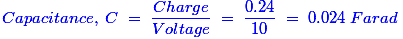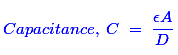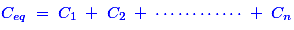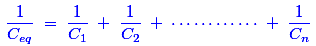Sunday, October 1, 2023

# Quick Quiz: Capacitor and Capacitance

#### Go on, take the challenge and share your score!

1. A capacitor stores 0.24 coulombs at 10 volts. Its capacitance is

a) 0.024 F.

b) 0.12 F.

c) 0.60 F.

d) 0.80 F

2. If the sheet of a backelite is inserted between the plates of an air capacitor, the capacitance will

a) decrease.

b) increase.

c) becomes zero.

d) remains unchanged.

3. The capacitance of a capacitor is not affected by

a) distance between plates.

b) area of plates.

c) thickness of plate.

d) all of them.

4. In a capacitor the electric charge is stored in

a) metal plates.

b) dielectric.

c) both of (A) and (B).

d) none of above.

5. Three capacitors each of capacity C are given. The resultant capacity (2/3) C can obtained by using them

a) all in series.

b) all in parallel.

c) two in parallel and third in series with this combination.

d) two in series and third in parallel across this combination.

6. The sparking between two electrical contacts can be reduced by inserting a

a) capacitor in parallel with contacts.

b) capacitor in series with each contacts.

c) resistance in line.

d) none of above.

7. In a capacitive circuit

a) a steady value of applied voltage causes discharge.

b) an increase in applied voltage make a capacitor charge.

c) decrease in applied voltage makes a capacitor charge.

d) none of above.

8. The inverse of capacitance is called

a) reluctance.

b) conductance.

c) susceptance.

d) elastance.

9. In a capacitor, the electrcal energy is stored in

a) magnetic field.

b) electric field.

c) both of (A) and (A).

d) none of above

10. Internal heating of a capacitor is usually attributed to

a) electron movement.

b) leakage reisitance.

c) dielectric charge.

d) plate vibration.

1. a)

We know that the capacitance is the ratio of charge and voltage. So,2. b)

Any electric charge produced by applied voltage and kept in bounds by an insulator (dielectric) that creates a dielectric field. Basically, Bakelite is a dielectric material (or) insulator and it has more dielectric strength than air medium. Due to the presence of this material in between the plates of an air capacitor, this can create the more dielectric field. Hence, the production of electric charge is high and thereby the capacitance value increases.

3. c)

The capacitance for any pair of separated conductors can be found with the below given expression:Where, E – Permittivity of dielectric,

A – Area of the plates,

D – Distance between the plates.

So, the distance and area could affect the changes in the capacitance of a capacitor. Hence, thickness of plate does not affect the capacitance value.

4. b)

In capacitors, the metal plates are used to connect a voltage source in between the dielectric medium. Hence, the electric charge is produced in due to the presence of dielectric medium and it is stored in that dielectric medium itself.

5. c)

We know that the capacitance value for parallel connected capacitor isand for series connected capacitors isHere, three capacitors have same value. Then, that the capacitance value for two parallel connected capacitor is ( C1+ C2 ) = (C + C ) = 2C. Now, this parallel connected combination is connected with one series connected capacitor. Hence, the final value of capacitance is6. b)

An arc between two electrodes can be initiated by ionization of atmospheric air. If we connect a capacitor across the contacts of the electrodes, there is a chance to make a short circuit by capacitor. Hence, there is no air gap between contacts and thereby sparking can be reduced.

7. b)

A charging current will flow into the capacitor that opposing any changes to the voltage at a rate and it is equal to the rate of change of the electrical charge on the plates. Hence, if voltage increases then according to that voltage, production of electric charge also increases.

8. d)

The elastance creates the voltage across a capacitor after accepting an electric charge of 1 coulomb; it is the reciprocal of the farad. The unit of elastance is the Daraf, but is not recognized by SI.

9. b)

The energy stored in the capacitor is stored as an electric field and the interesting things is the capacitor as a device which either stores energy in, or extracts energy from, an electric field.

10. b)

A real capacitor has a leakage resistance internally. It can be said as a large resistance in parallel with capacitor. While the leakage current flowing in it, and then I2R loss is produced due to that leakage resistance. Hence, the internal loss happens due to the leakage power loss inside the capacitor.

Courtesy: electrical4u

#### 1 COMMENT

1.Atharva

Can you explain why the dielectric is the real seat of charge and not the metal plates